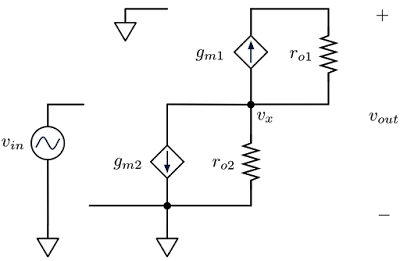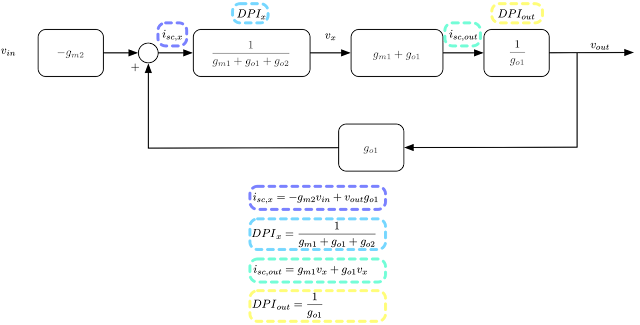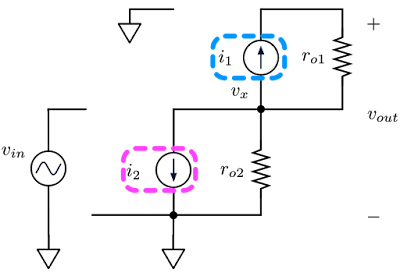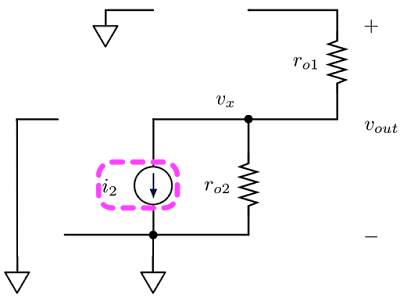## Tuesday, July 18, 2017

### How to Make Meringue: Cascode Edition!

Have you ever made meringues? I'm a big fan of pavlova (AKA meringue cake) but unfortunately for me, pavlova isn't exactly popular in CA. Hence, I've gone through several meringue recipes. They all differ drastically from how to introduce sugar, what speed to beat, what temp to bake (and the duration...sigh), etc. And of course, *everyone* claims their recipe is "the best and most fool-proof" (hah!). I've come to the conclusion that everyone has deeply ingrained, arbitrary superstitions regarding meringue (then again, I still believe in my lucky exam pencil, so who am I to judge?). All jokes aside, the only thing that really matters is the beaten egg mixture doesn't slip out of the bowl when flipped turned upside down...(at least that's my fool-proof superstition 😆).

Likewise, the methodology behind the construction of feedback diagrams is as idiosyncratic (if not more so) than meringue. Everyone has their own peculiar feedback recipe and it's all really the same thing. Granted, I do prefer the Driving-Point-Impedance (DPI), short-circuit current. signal-flow graph (SFG) method outlined by Agustin Ochoa, but again this is more of a matter of personal taste. I specifically like Ochoa's process in that it naturally gives additional circuit information (ex: output impedance, example later in post).

Let's take a very familiar circuit for demonstration. What's more beloved than the reliable cascode (Fig 1)?Fig. 1 - CascodeFig. 2 - Cascode Small-Signal Model
Let's start with the tried and true small signal model (Fig. 2) and algebra method. This gives us our voltage gain (Eqn. 1)Eqn. 1 (meh it's a set, oh well)

If we're judging methodologies in terms of speed and simplicity, the normal algebra method wins (for this specific case). It's easy, but it may not give other information (which is fine if all that's asked is voltage gain).

Now let's look at Ochoa's method (I super recommend his book).

First, we'll grab the short circuit currents. We set all potential voltage nodes as "independent voltage sources" and short the voltage source of interest (we are getting the short-circuit current after all). Then we grab the DPI by shorting all the other voltage sources and opening our node of interest (hence the driving point impedance part). Note: this all works because for each node we're grabbing: 1) short-circuit current *and* 2) "open circuit" (really driving point) impedance which gives us a voltage for each node (hence the whole fake independent voltage source for each node). We then methodically move through all the nodes until we have hit the output node. Think of it as superposition mayhem. For more info, I strongly suggest Ochoa's book mentioned above.

Now we look at our first node: $v_{x}$. For our short circuit current, we set $v_{out}$ as a source, then short $v_{x}$ (Fig. 3). This gives us the following short circuit current (Eqn. 2, note we short across $r_{o2}$, hence no contribution). Also no contribution from $g_{m1}$ because we've shorted $v_{x}$.Fig. 3Eqn. 2
For DPI (we are searching for "$Z_{x}$"), we short all sources and open our node of interest. This leaves $v_{x}$ open while all the other voltage sources are shorted (Fig. 4). $g_{m2}$ is open because the input voltage is shorted. This reduces to $g_{m1}$ and $r_{o1}$ and $r_{o2}$ all in parallel, leaving us the DPI of Eqn. 3.Fig. 4Eqn. 3
For $v_{out}$ short-circuit current, we set $v_{x}$ as an independent source and short $v_{out}$ (Fig. 5). The short circuit current is equal to the contribution from $\left( g_{m1}+g_{o1} \right) v_{x}$.Fig. 5Eqn. 4
The DPI for $v_{out}$ is simply $r_{o1}$ since $r_{o2}$ is shorted (from $v_{x}$) (Eqn. 5). Both $g_{m1}$ and $g_{m2}$ are open since both $v_{in}$ and $v_{x}$ are shorted to GND (Fig. 6).Fig. 6Eqn. 5
Now that we have a complete set of short-circuit and DPI equations, let's put together our feedback graph. Combing equations 2 through 5, we get the following.Fig. 7Eqn. 6

Hooray, we get our voltage gain (Eqn. 6)!!!

Now a few interesting things to note from Fig. 7. First off, we actually get the same voltage gain as algebra method because we have positive feedback from the output voltage (Fig. 7). As we look back on the original small signal model, this makes some sense. Resistors are bi-directional, hence there is some "backwards-leaking" current from $v_{out}$ to the $v_{x}$ node (which ends up mingling with the $-g_{m2}*v_{in}$). Second off, we can calculate output resistance easily from Fig. 7. In fact, Fig. 7's output impedance matches the algebraic solution (Eqn. 7 derived from Fig. 7 is the same result as the traditional algebra).Eqn. 7
The third cool part is let's say there's some voltage noise coupling to $v_{x}$ from another trace in the circuit. With the overall block diagram, we can see how the coupled noise to $v_{x}$ would look on the output by calculating $\frac { v_{ out } }{ v_{ x } }$. We could also introduce current noise sources at the various short-circuit currents to simulate shot noise. Heck, you can add noise sources where ever desired and see the effect on the output. The real magic is the feedback diagram enables noise modeling.

Equally important (which I haven't listed here) is if this exercise is re-done with reactive components (mostly caps), it should become obvious which caps affect bandwidth/stability the most.

Now...there is a third method to calculate voltage gain via *another* feedback graph technique. I haven't found a formal name for this technique other than "‘Fake Label’ Circuit-Analysis Trick" from Ultra Low Power Bioelectronics.

I don't particularly like this method because the diagram produced isn't guaranteed to carry more information other than the *specific* output/input requested. Why? Ochoa's method intentionally dissects the circuit into short-circuit current, driving point impedance, and voltage sub-blocks ($i_{sc}*DPI = v_{node}$). This is great in that we can easily get impedance, $\frac { i_{ out } }{ i_{ in } }$, and $\frac { v_{ out } }{ v_{ in } }$ naturally by looking at the diagram because it's already intentionally broken into current, impedance, voltage. The "Fake Label" method instead takes all the dependent sources, makes them fake independent sources, and re-builds a diagram by turning each source on one at a time. While the overall transfer function (as in output/input only) might be accurate, the "Fake Label" feedback diagram won't be in current to impedance to voltage blocks, so information from intermediate nodes within the overall diagram may not be accurate/present. Thus with "Fake Label", you end up drawing different diagrams for different specified transfer functions (like the examples below with voltage gain and output impedance).

However, the useful part of "Fake Label" is that it's more simple than DPI/short-circuit current - we're only looking at one source at a time. For larger circuits, I can see using "Fake Label" over Ochoa's.

For example, let's look at "Fake Label" for cascode's voltage gain.

First, we would turn all the $g_{m}$ dependent sources into independent current sources. Our control variables will be $v_{x}$ and $v_{out}$.Fig. 8

Now we step through turning on one source at a time.

If $v_{in}$ were on but the other two current sources off (i.e. open), $v_{x}$ and $v_{out}$ see no contribution. Hence nothing to write about here.

If $g_{m2}$ (called $i_{2}$) were on and $v_{in}$ were off (i.e. short to ground) while $g_{m1}$ were off (open), then we see the following.Fig. 9Eqn. 8

If $g_{m1}$ (called $i_{1}$) were on and $v_{in}$ were off and while $g_{m2}$ were off, then we see the following.Fig. 10Eqn. 9

Eqns 8-9 create Fig. 11 (which has the correct voltage gain). However, this diagram doesn't describe the output impedance (and I wouldn't use Fig. 11's $\frac { v_{ out } }{ v_{ x } }$ either since the intermediate nodes aren't meticulously split into current and DPI). Again, we haven't re-conditioned the system into short-circuit current, driving point impedance, and voltage sub-blocks (a sub-block for each node) but rather look at the aggregate contribution to a node via several "independent" sources. Because the processes are different (which leads to different equations as well), we get different block diagrams.Fig. 11

This means that if we want output impedance (via "Fake Label"), we'll have to construct another diagram... Sources will be $v_{t}$ (previously output voltage) and $i_{1}$. $i_{2}$ disappears because the input is grounded in order to calculate output impedance. Control variables are $i_{t}$ and $v_{x}$.Fig. 12

If $i_{1}$ is on and $v_{t}$ is off, then we see the following.Fig. 13Eqn. 10

If $v_{t}$ is on and $i_{1}$ is off, then we see the following.Fig. 14Eqn. 11

Putting this all this together leads to the following (Fig. 15). The overall transfer function is equal to the inverse of the output impedance!Fig. 15
As we've seen, there's a lot of ways bake meringue. In terms of pure output/input transfer function, they all end up with the same result. Depending on what you're looking for, one way may be better than others (Table 1). Again, it's a matter of personal taste (along with objective), but I would definitely take a look at both Feedback in Analog Circuits by Agustin Ochoa and Ultra Low Power Bioelectronics by Rahul Sarpeshkar if this is interesting. Seems like classical feedback is somewhat of a dying art nowadays (boo), but the intuition is still fun and it's always good to expand one's palette, even if it all makes the same meringue. Hah!Table. 1